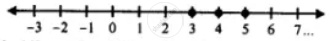# For the Following Inequation, Represent the Solution on a Number Line : 2(4 - 3x) ≤ 4(X - 5), X ∈ W - Mathematics

Sum

For the following inequation, represent the solution on a number line :

2(4 - 3x) ≤ 4(x - 5), x ∈ W

#### Solution

2(4 - 3x) ≤ 4(x - 5), x ∈ W

8 - 6x ≤ - 20 - 8

⇒ -6x - 4x ≤ - 20 - 8

⇒ -10x ≤ - 28

⇒  10x ≥ 28

⇒ x ≥ 28/10

⇒ x ≥ 2.8

∴ x = {3, 4, 5, ....}Concept: Operation on of Whole Number on Number Line
Is there an error in this question or solution?

#### APPEARS IN

Selina Concise Mathematics Class 8 ICSE
Chapter 15 Linear Inequations
Exercise 15 (B) | Q 13.3 | Page 175